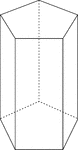### Pentagonal Prism

Illustration of a right pentagonal prism with regular pentagons for bases and rectangular faces. The…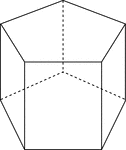### Pentagonal Prism

Illustration of a right pentagonal prism with regular pentagons for bases and rectangular faces. The…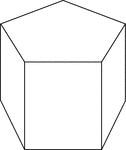### Pentagonal Prism

Illustration of a right pentagonal prism with regular pentagons for bases and rectangular faces. The…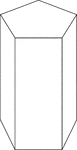### Pentagonal Prism

Illustration of a right pentagonal prism with regular pentagons for bases and rectangular faces. The…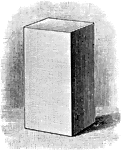### Right Prism

Illustration of a right prism - a prism whose lateral edges are perpendicular to its bases.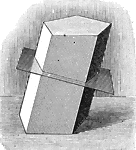### Right Section of Prism

Illustration of a right section of a prism.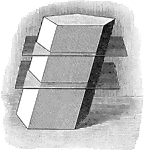### Sections of Prism

Illustration of the sections of a prism made by parallel planes cutting all the lateral edges into equal…### Sections of Prism

Illustration of the sections of a prism made by parallel planes cutting all the lateral edges into equal…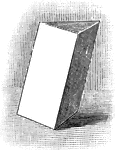### Triangular Prism

Illustration of a triangular prism - a prism whose base is a triangle.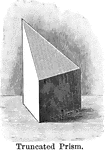### Truncated Prism

Illustration of a truncated prism - the part of a prism included between the base and a section made…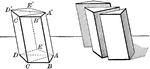### Prism Showing Volume

Illustration of a prism used to demonstrate that the volume of any prism is equal to the product of…### 2 Octagonal Prisms

Illustration of 2 right octagonal prisms with congruent bases, but different heights. The height of…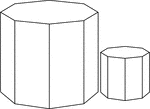### 2 Similar Octagonal Prisms

Illustration of 2 Similar right octagonal prisms. The height and length of the edges of the smaller…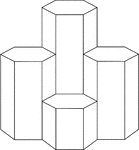### 4 Hexagonal Prisms

A cluster of 4 right hexagonal prisms with congruent bases, but varying heights.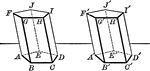### Equal Prisms

Illustration showing two prisms are equal if the three faces which include a trihedral angle of the…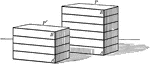### Rectangular prisms

Illustration of 2 parallelopipeds/rectangular prisms.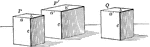### Rectangular prisms

Illustration of 3 parallelopipeds/rectangular prisms.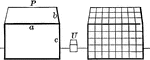### Rectangular Prisms Showing Volume

Illustration of 2 rectangular prism used to show volume.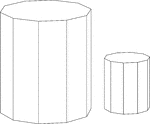### Similar Decagonal Prisms

Illustration of 2 similar right decagonal prisms. Both have regular decagons for bases and rectangular…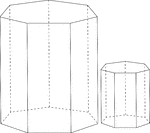### Similar Heptagonal/Septagonal Prisms

Illustration of 2 similar right heptagonal/septagonal prisms. Both have regular heptagons/septagons…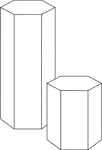### Similar Hexagonal Prisms

Illustration of 2 similar right hexagonal prisms. The height of the prism and length of the side of…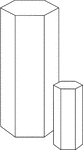### Similar Hexagonal Prisms

Illustration of 2 similar right hexagonal prisms. The height of the prism and length of the side of…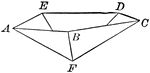### Inverted Pentagonal Pyramid

Illustration of an inverted pentagonal pyramid. It is pyramid with a regular pentagon for its base.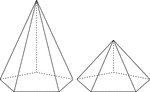### 2 Right Pentagonal Pyramids

Illustration of 2 right pentagonal pyramids with hidden edges shown. The pentagonal bases are congruent,…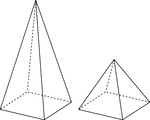### 2 Right Rectangular Pyramids

Illustration of 2 right rectangular pyramids with hidden edges shown. The rectangular bases are congruent,…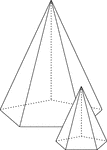### Similar Pentagonal Pyramids

Illustration of 2 similar right pentagonal pyramids with hidden edges shown. The height of the pyramid…### Bottom Half of a Regular Dodecahedron

Illustration of a the bottom half of a regular dodecahedron. A regular dodecahedron is a polyhedron…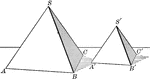### Similar Tetrahedrons

The volumes of two similar tetrahedrons are to each other as the cubes of their homologous (corresponding)…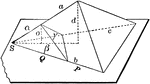### Two Proportional Tetrahedrons

Diagram used to prove the theorem: "Two tetrahedrons having a trihedral angle in each equal, are to…